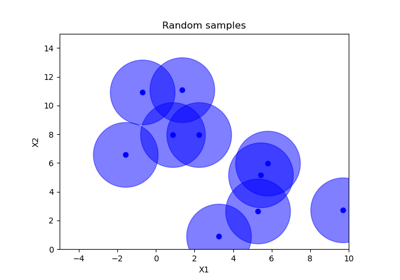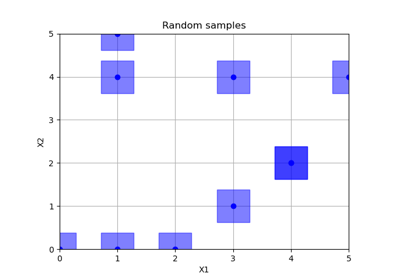# skopt.sampler.Halton¶

class skopt.sampler.Halton(min_skip=-1, max_skip=-1, primes=None)[source][source]

Creates Halton sequence samples. In statistics, Halton sequences are sequences used to generate points in space for numerical methods such as Monte Carlo simulations. Although these sequences are deterministic, they are of low discrepancy, that is, appear to be random for many purposes. They were first introduced in 1960 and are an example of a quasi-random number sequence. They generalise the one-dimensional van der Corput sequences.

For dim == 1 the sequence falls back to Van Der Corput sequence.

Parameters
min_skipint

minimum skipped seed number. When min_skip != max_skip a random number is picked.

max_skipint

maximum skipped seed number. When min_skip != max_skip a random number is picked.

primestuple, default=None

The (non-)prime base to calculate values along each axis. If empty or None, growing prime values starting from 2 will be used.

Methods

 generate(dimensions, n_samples[, random_state]) Creates samples from Halton set. set_params(**params) Set the parameters of this initial point generator.
__init__(min_skip=-1, max_skip=-1, primes=None)[source][source]

Initialize self. See help(type(self)) for accurate signature.

generate(dimensions, n_samples, random_state=None)[source][source]

Creates samples from Halton set.

Parameters
dimensionslist, shape (n_dims,)

List of search space dimensions. Each search dimension can be defined either as

• a (lower_bound, upper_bound) tuple (for Real or Integer dimensions),

• a (lower_bound, upper_bound, "prior") tuple (for Real dimensions),

• as a list of categories (for Categorical dimensions), or

• an instance of a Dimension object (Real, Integer or Categorical).

n_samplesint

The order of the Halton sequence. Defines the number of samples.

random_stateint, RandomState instance, or None (default)

Set random state to something other than None for reproducible results.

Returns
np.array, shape=(n_dim, n_samples)

Halton set

set_params(**params)[source]

Set the parameters of this initial point generator.

Parameters
**paramsdict

Generator parameters.

Returns
——-
selfobject

Generator instance.

## Examples using skopt.sampler.Halton¶